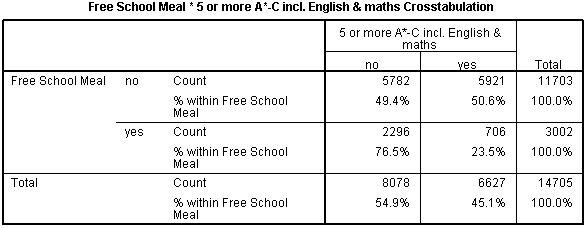# Using Statistical Regression Methods in Education Research# Logistic Regression Module Quiz A

Your total score is :0 / 9

Below is a 9 question quiz (a ‘quizlet’, if you like) to help you to familiarise yourself with all of these confusing new terms that we have introduced. Click VIEW FEEDBACK when you’re ready to see the answers. When you want to move on just click EXIT QUIZ. Enjoy!

2. Take a look at the following crosstabulation. It shows the relationship between whether a student receives a minimum of 5 A*-C grades at age 16 (fiveem) and whether or not they are eligible to receive free school meals from the government (FSM):Which of the following statements are true (click all that apply)?

3. Four equally matched horses (A-D) run in a race. Supposing that all horses are equally likely to win, what is the probability that horse A will be victorious?

4. Continuing with our equestrian theme, what are the odds that the aforementioned horse A will win?

5. The probability of a student in a statistics class passing a horrible test is 0.75. What are the odds of a student passing this test compared to not passing the test?

6. The following statements are about logits and odds ratios. Drag each statement under the correct category.
 Statement Logits Odds Ratio Symetrical around '0' Sign (+ or -) dependent on 'base' category Linear relationship with the explanatory variables Change in odds of outcome associated with a one unit change in explanatory variable Minimum value of 0

7. What does the exponent of the regression coefficient, Exp(B), represent in a logistic regression model?

9. Suppose that in our sample, following a logistic regression analysis, the odds for girls of having a positive attitude to school were 1.25. What proportion of girls would be expected to have a positive attitude?

Privacy statement
This quiz is anonymous. No data which personally identifies you is collected on the quiz, and the data you provide is used solely to help us improve the delivery of our courses.
Page contact: Feedback to ReStore team Last revised: Mon 7 Mar 2011